# Reverse Colors

## Recommended Posts

Hi,

I was trying to create some medusa with the Rosace plugin like this: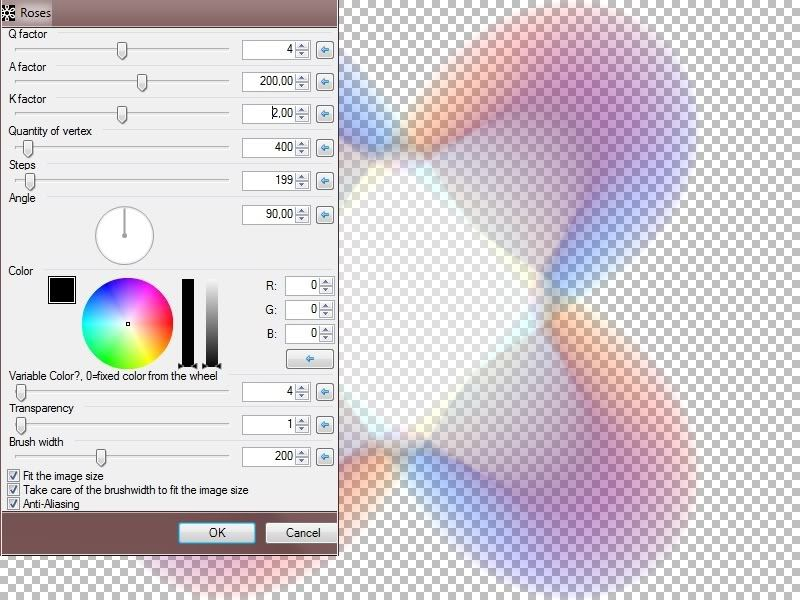Using a very low transparency with a large brushwidth it's making some smooth colorfull shapes.

Then I thought it would be nice to have the edge less transparent than the middle...

This is easy with CodeLab to do that. And then why not do the same per chanel...

This is the result with the UI: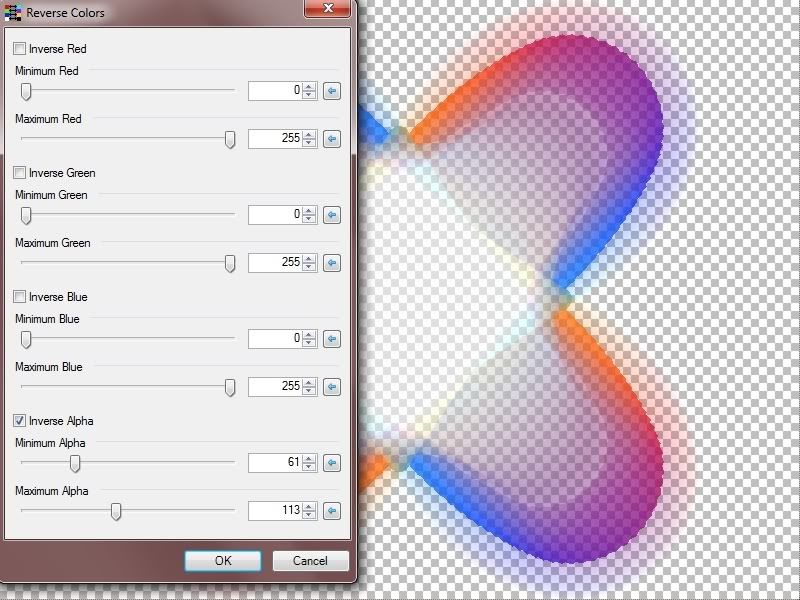The code:

```#region UICode
bool Amount1 = false; // [0,1] Inverse Red
int Amount2 = 0; // [0,255] Minimum Red
int Amount3 = 255; // [0,255] Maximum Red

bool Amount4 = false; // [0,1] Inverse Green
int Amount5 = 0; // [0,255] Minimum Green
int Amount6 = 255; // [0,255] Maximum Green

bool Amount7 = false; // [0,1] Inverse Blue
int Amount8 = 0; // [0,255] Minimum Blue
int Amount9 = 255; // [0,255] Maximum Blue

bool Amount10= true; // [0,1] Inverse Alpha
int Amount11= 1; // [0,255] Minimum Alpha
int Amount12 = 254; // [0,255] Maximum Alpha
#endregion

void Render(Surface dst, Surface src, Rectangle rect)
{
// Delete any of these lines you don't need
Rectangle selection = this.EnvironmentParameters.GetSelection(src.Bounds).GetBoundsInt();
int SelL = selection.Left;
int SelR = selection.Right;
int SelB = selection.Bottom;
int SelT = selection.Top;
int CenterX = ((SelR - SelL) / 2) + SelL;
int CenterY = ((SelB - SelT) / 2) + SelT;

ColorBgra PrimaryColor = (ColorBgra)EnvironmentParameters.PrimaryColor;
ColorBgra SecondaryColor = (ColorBgra)EnvironmentParameters.SecondaryColor;
int BrushWidth = (int)EnvironmentParameters.BrushWidth;
int r,g,b,a;

ColorBgra CurrentPixel;
for (int y = rect.Top; y < rect.Bottom; y++)
{
for (int x = rect.Left; x < rect.Right; x++)
{
CurrentPixel = src[x,y];
if(Amount1)
{
r = CurrentPixel.R;
if (r >= Amount2 && r <= Amount3) CurrentPixel.R = (byte)(255 - r);
}
if(Amount4)
{
g = CurrentPixel.G;
if (g >= Amount5 && g <= Amount6) CurrentPixel.G = (byte)(255 - g);
}
if(Amount7)
{
b = CurrentPixel.B;
if (b >= Amount8 && b <= Amount9) CurrentPixel.B = (byte)(255 - ;
}
if(Amount10)
{
a = CurrentPixel.A;
if (a >= Amount11 && a <= Amount12) CurrentPixel.A = (byte)(255 - a);
}
dst[x,y] = CurrentPixel;
}
}
}
```

>>>>>>>>>>

Here is the zip for the DLL

>>>>>>>>>>

Then my question are :

1. Is this too similar to an existing plugin (as I could have miss some...)

2. What do you think of it?

This is mainly the medula idea (WIP)...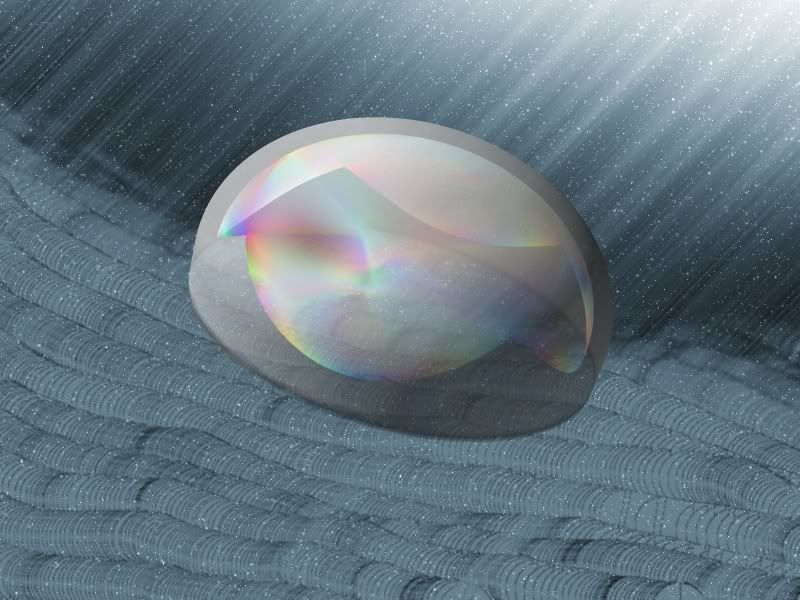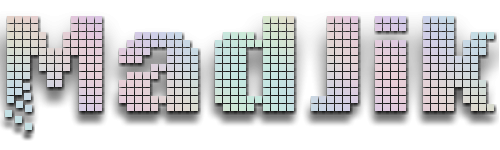My DeviantArt | My Pictorium | My Plugins | Donate via Paypal

##### Share on other sites

The plugin could be very useful since it gives you the choice of which colours to invert.Knowledge is no burden to carry.

April Jones, 2012

##### Share on other sites

I really like the plugin, I say add it. I've not seen something that's too similar.

offtopic:

In your image, you might want to make the lighting of the 3D shape face the lighting coming down from the right side. The fact that the light looks like it's coming from the other way looks weird, lol.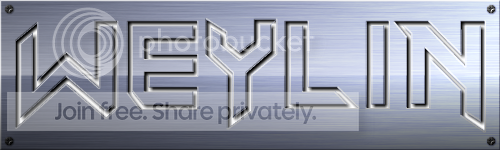## Join the conversation

You can post now and register later. If you have an account, sign in now to post with your account.×   Pasted as rich text.   Paste as plain text instead

Only 75 emoji are allowed.

×   Your previous content has been restored.   Clear editor

×   You cannot paste images directly. Upload or insert images from URL.

×
×